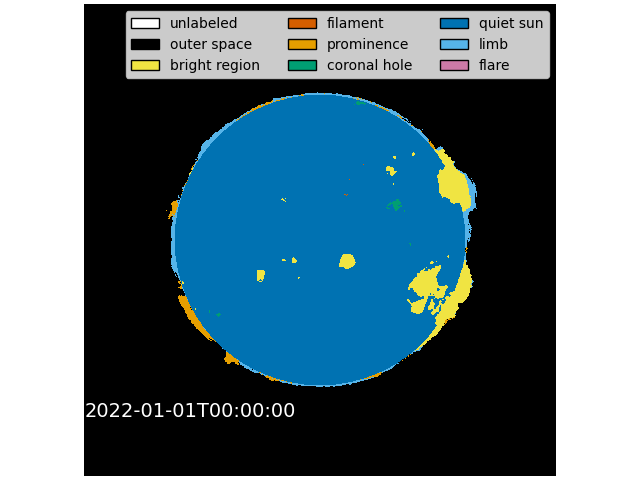# Plotting a Level 2 SUVI Thematic Map#

This example shows how to read a SUVI L2 Thematic Map FITS file and plot it.

SUVI L2 Thematic Maps are recognized by pattern in the filename, i.e. they contain “-l2-thmap”.

```import matplotlib.pyplot as plt
from matplotlib.colors import ListedColormap
from matplotlib.patches import Patch

from astropy.io import fits

from sunkit_instruments.suvi._variables import SOLAR_CLASS_NAME, SOLAR_COLORS
```

We start with getting the data. This is done by downloading the data from `data.ngdc.noaa.gov`.

In this case, we will use requests as to keep this example self contained but using your browser will also work.

```url = [
"https://data.ngdc.noaa.gov/platforms/solar-space-observing-satellites/goes/goes16/l2/data/suvi-l2-thmap/2022/01/01/dr_suvi-l2-thmap_g16_s20220101T000000Z_e20220101T000400Z_v1-0-2.fits"
]
```

First let’s read a SUVI L2 Thematic Map FITS file.

```with fits.open(file) as hdu:
thmap_data = hdu.data
```

Now we will plot it.

```# Here we have some logic to get the correct color map for the SUVI L2 Thematic Map.
colortable = [
SOLAR_COLORS[SOLAR_CLASS_NAME[i]] if i in SOLAR_CLASS_NAME else "black"
for i in range(max(list(SOLAR_CLASS_NAME.keys())) + 1)
]
cmap = ListedColormap(colortable)

# Now the plotting code.
fig, ax = plt.subplots(constrained_layout=True)
ax.imshow(
thmap_data,
origin="lower",
cmap=cmap,
vmin=-1,
vmax=len(colortable),
interpolation="none",
)
ax.set_axis_off()
ax.text(0, 158, time_stamp, fontsize=14, color="white")
legend_elements = [
Patch(facecolor=color, edgecolor="black", label=label.replace("_", " "))
for label, color in SOLAR_COLORS.items()
]
ax.legend(
handles=legend_elements,
loc="upper right",
ncol=3,
fancybox=True,